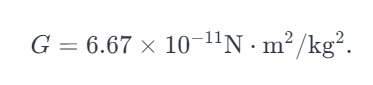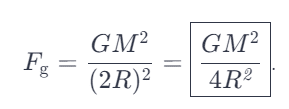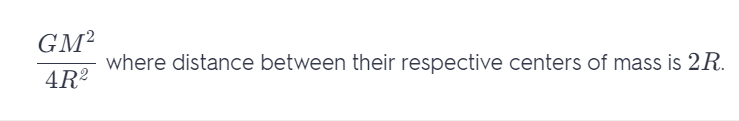# Two Uniform Spheres, Each With Mass M And Radius R, Touch One Another.

We thoroughly check each answer to a question to provide you with the most correct answers. Found a mistake? Let us know about it through the REPORT button at the bottom of the page.

Two uniform spheres, each with mass M and radius R, touch each other. What is the magnitude of their gravitational force of attraction?

F = GMM/(2R)² = GM²/4R²

defines the magnitude of attractive gravitational force between two bodies of masses m1 and m2 when they are separated by a distance r.

r represents the {\bf separation of the centers of mass } of the bodies.

(This detail can be extrapolated from the derivation of equation 13.2, describing the weight of a small body on the surface of the earth. The radius of the earth is accented here, being the separation of the body to the center of mass of the earth. The “radius” of the small body is neglected as it is relatively small compared to the earth’s.)m1 = m2 = M

The separation of the centers of the touching spheres (centers of their masses) is r = 2R.

The magnitude of the gravitational attraction between them is